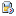# Software1DFD_DS
The Fortran95 Computer Code for Finite-Difference Numerical Generation and Simulation of a 1D Seismic Wavefield in a 1D Heterogeneous Viscoelastic Medium Using the Displacement-Stress Staggered-Grid Finite-Difference Scheme1DFD_DVS
The Fortran95 Computer Code for Finite-Difference Numerical Generation and Simulation of a 1D Seismic Wavefield in a 1D Heterogeneous Viscoelastic Medium Using the Displacement-Velocity-Stress Staggered-Grid Finite-Difference Scheme1DFD_VS
The Fortran95 Computer Code for Finite-Difference Numerical Generation and Simulation of a 1D Seismic Wavefield in a 1D Heterogeneous Viscoelastic Medium Using the Velocity-Stress Staggered-Grid Finite-Difference Scheme2DSPEC-2.0
A parallel/serial 2d spectral element code for wave propagation and rupture dynamics3DFD_DVS
The program is designed for computation of seismic wavefields in 3D heterogeneous surface geological structures with planar free surface due to surface and near-surface point doublecouple sourcesAnalytical_Anelastic
An analytical solution to anelastic wave propagation in a homogenous mediumAnalytical_Poroelastic
An analytical solution to poroelastic wave propagation in a homogenous mediumCouplage
Modelling of propagation of surface waves in 3D structures by mode coupling methodDirect Solution Method
DSM software for calculating full wave synthetics in a laterally homogeneous spherically symmetric earth modelEX2DDIR
Exact 2D Response For VACUUM/ELASTIC Interface, Directional Source (Lamb's Problem)EX2DELEL
Exact 2D Response For ACOUSTIC/ELASTIC or ELASTIC/ELASTIC Interface, Compressional SourceEX2DVAEL
Exact 2D Response for Vacuum/Elastic Interface, Compressional Source (Garvin's Problem)FD3S
Finite-difference solver of the elastic wave equation in a spherical section. FD3S allows to model seismic wave attenuation as well as anisotropy with radial symmetry axis. The finite difference scheme is of fourth order in space and of second order in time. Arbitrarily complex Earth models can easily be included.FD3S(AD)
FD3S(AD) is an extension of FD3S (see above) that allows to compute the derivative of an objective functional defined on a recorded seismic wavefield with respect to the model parameters. The computation of the derivative is based on the adjoint method.Fd2d
This is a very simple training code for Finite Differences (FD) in 2D.GEMINI
Calculation of synthetic seismograms for global, spherically symmetric media based in direct evaluation of Green's functionsGar6more 3D
Analytical Solutions of Waves Propagation Problems in Stratified Media in 3D.Gar6more 2D
Analytical Solutions of Waves Propagation Problems in Stratified Media in 2D.HS3M_4M
Fault zone waves: Analytical solutionsISOLA moment tensor retrieval software package
Fortran code ISOLA to retrieve isolated asperities from regional or local waveforms based on multiple-point source representation and iterative deconvolution.LD_BIMAT_2D
Calculates the 2 components of the 2D wavefield generated by a line dislocation at the interface between two different elastic solids.Normal modes
Normal-mode based computation of seismograms for spherically symmetric Earth modelsPD_BIMAT
Calculates the 3 components wavefield generated by a point dislocation at the interface between two different elastic solids.Ps2d
This is a very simple code for elastic wave simulation in 2D using a Pseudo-Spectral Fourier methodRay theory
Ray-theoretical approach to the calculation of synthetic seismograms in global Earth models.Reflectivity method
Code for calculating the response of a stratified set of uniform solid layers to excitation by a point moment tensor source using the Reflectivity Method (RM).SHaxi
SHaxi performs elastic and anelastic global SH wave propagation in the Earth's mantle for axi-symmetric geometries.Shansyn
Package of Spherical Harmonics ANalysis and SYNthesis tools for UNIX/GMT environments. Required to plot model coefficients downloaded from Becker and Boschi's database.SismoVi
It is an interactive application for the pre- & post-processing of data for seismic wave modelling. Three integrated modules ( GeomodVi, MeshVi, DataVi ) allow for an easy preparation, processing and visualisation of such data.Surface Wave Ray Tracing with Azimuthal Anisotropy
surface wave ray tracing as described by Boschi and Woodhouse GJI 2006TI_analytical_3D
Analytical solution for 3D anelastic (transversely isotropic) homogeneous material due to a point force source.Time-frequency Misfits
The Fortran95 program TF-MISFITS is designed for computation of time-frequency misfits between tested and reference seismogramsUnderstanding seismic radiation patterns (tutorial)
The Matlab routine anaseis.m shows the 3D radiation pattern and the corresponding seismograms for various source types.VOLPIS
Volpis is a Fortran code for source inversion in volcano seismology. The code can be used to retrieve the time-dependent source components (moment tensor and single forces) of volcanic events.Vphase
Calculation of phase velocity dispersion curvesmembraneSphere
Software package membraneSphere simulates membrane waves over the whole globe.sem2dpack
A spectral element package for 2D wave propagation and earthquake rupture dynamics